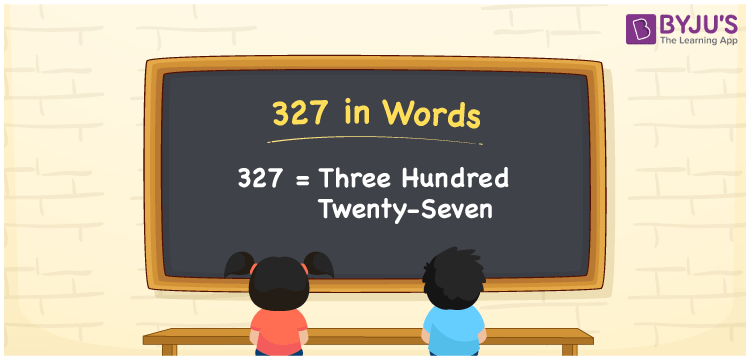# 327 in Words

327 in words is written as Three hundred twenty-seven. Thus, the word form or number name of 327 is given by Three hundred twenty-seven. This word form is used to verbally express or convey the number to others. Also, we know that to convert three-digit numbers into word form, we require a place value chart. In this article, you will understand the conversion of the cardinal number 327 into words.

 327 in words Three hundred twenty-seven Three hundred twenty-seven in Numbers 327

## 327 in English Words

We generally write numbers in words using the English alphabet. So, we spell 327 in English words as “Three hundred twenty-seven”.## How to Write 327 in Words?

The number 327 has three digits so, let’s make a place value chart for 327 to define the place values for all the digits.

 Hundreds Tens Ones 3 2 7

Here, ones = 7, tens = 2, hundreds = 3

These can be written as:

3 × Hundred + 2 × Ten + 7 × One

= 3 × 100 + 2 × 10 + 7 × 1

= 300 + 20 + 7

= Three hundred + Twenty + Seven

= Three hundred twenty-seven

Therefore, 327 in words = Three hundred twenty-seven.

327 is a natural number that precedes 328 and succeeds 326.

327 in words – Three hundred twenty-seven

Is 327 an even number? – No

Is 327 an odd number? – Yes

Is 327 a prime number? – No

Is 327 a composite number? – Yes

Is 327 a perfect square number? – No

Is 327 a perfect cube number? – No

## Frequently Asked Questions on 327 in Words

Q1

### How do you express 327 in word form?

We can express the number 327 in word form as Three hundred twenty-seven.
Q2

### How to write Rs. 327 in words on a cheque?

On a cheque, we generally write Rs. 327 in words as “Three hundred twenty-seven rupees only”.
Q3

### Write 327.040 in words.

We can write the decimal number 327.040 in words as Three hundred twenty-seven point zero four zero.